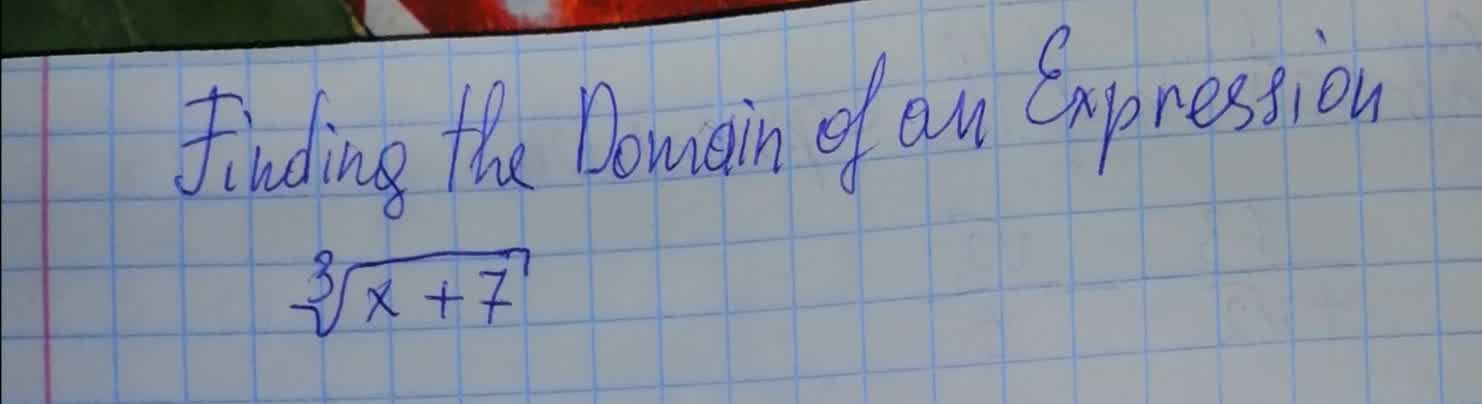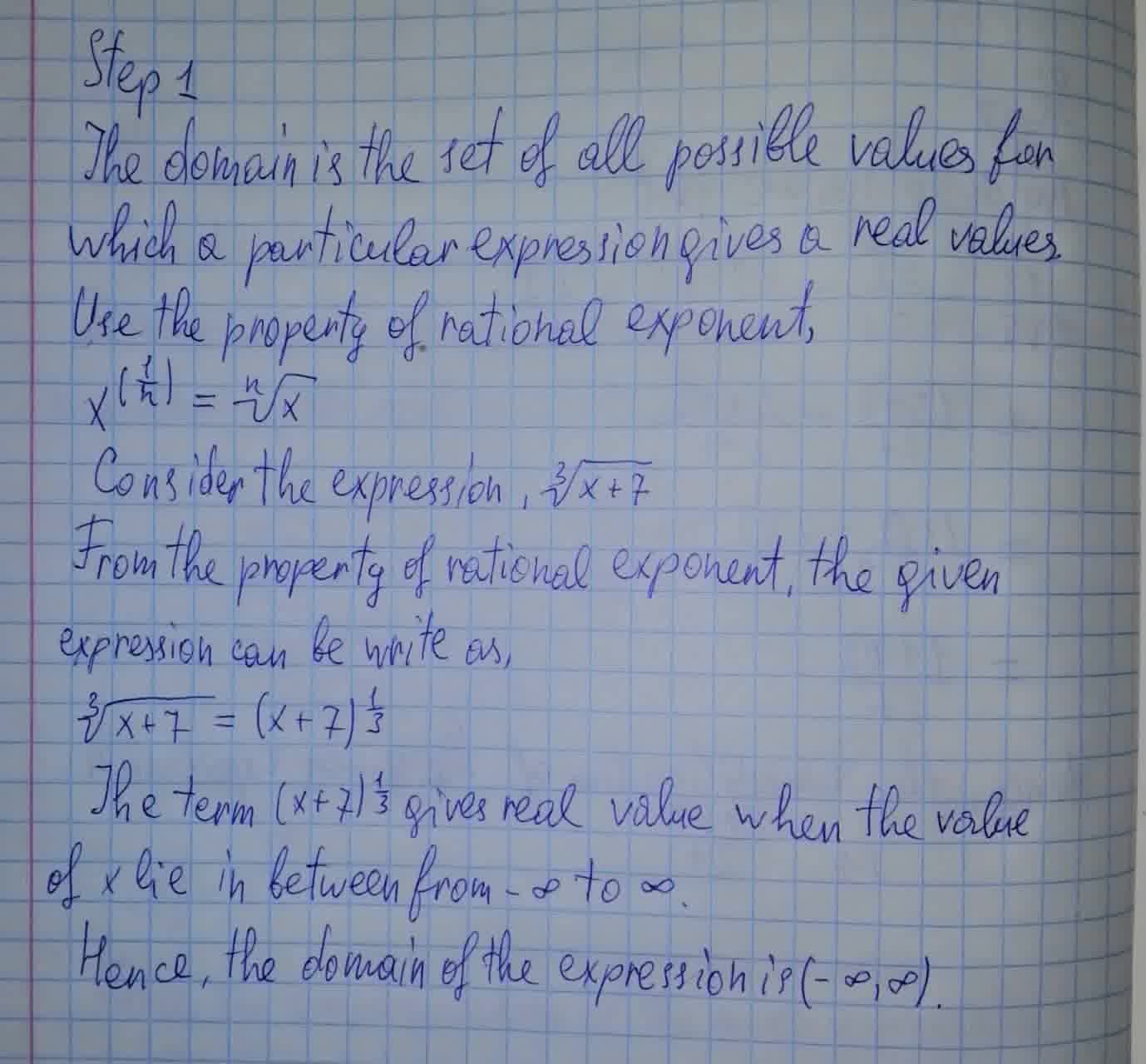permaneceerc

2021-08-10

To calculate : The domain of the expression $\sqrt{x+7}$Bella

The answer is given below in the photo2021-12-24

Step 1

The domain is the set of all possible values for which a particular expression gives a real values

Use the property of rational exponent,

${x}^{\left(\frac{1}{n}\right)}=\sqrt[n]{x}$

Consider the expression, $\sqrt{x+7}$

From the property of rational exponent, the given expression can be write as,

$\sqrt{x+7}=\left(x+7{\right)}^{\frac{1}{3}}$

The term $\left(x+7{\right)}^{\frac{1}{3}}$ gives real value when the value of x lie in between from $-\mathrm{\infty }$ to $\mathrm{\infty }$

Hence, the domain of the expression is $\left(-\mathrm{\infty },\mathrm{\infty }\right)$

Do you have a similar question?